# Weighted Average Algorithm

Weighted Average Algorithm is an algorithm for prediction with expert advice, one of the exponential weights algorithms. In the simplest case, the algorithm predicts a sequence of numbers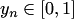using advice from a finite number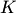of experts. The prediction space is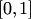. Each expert is initially assigned weight. Unlike Strong Aggregating Algorithm, the master's prediction is the weighted average of the experts' predictions, and this simplicity is the main advantage of Weighted Average Algorithm. It can be shown, that for the square-loss game (see loss function) the algorithm guarantees the inequality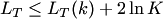for all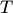and, where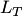is the loss suffered by master over the firsttrials, and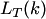is the loss suffered by theth expert over the firsttrials.

The Weighted Average Algorithm was introduced by Kivinen and Warmuth (1994), in particular for the square-loss game, log-loss game, and game with Hellinger loss. It was generalized for the case where output is multi-dimensional,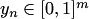.

The interesting fact about the Weighted Average Algorithm is that the first bound is derived for the case of non-mixable games, and it depends onas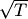. This fact can be a starting point to answer on the Better Loss Bounds for Mixable Games.

### Bibliography

• Jyrki Kivinen and Manfred K. Warmuth. Averaging expert predictions. In Paul Fischer and Hans U. Simon, editors, Computational Learning Theory: 4th European Conference, volume 1572 of Lecture Notes in Artificial Intelligence, pp. 153 - 157, Berlin, 1999. Springer.